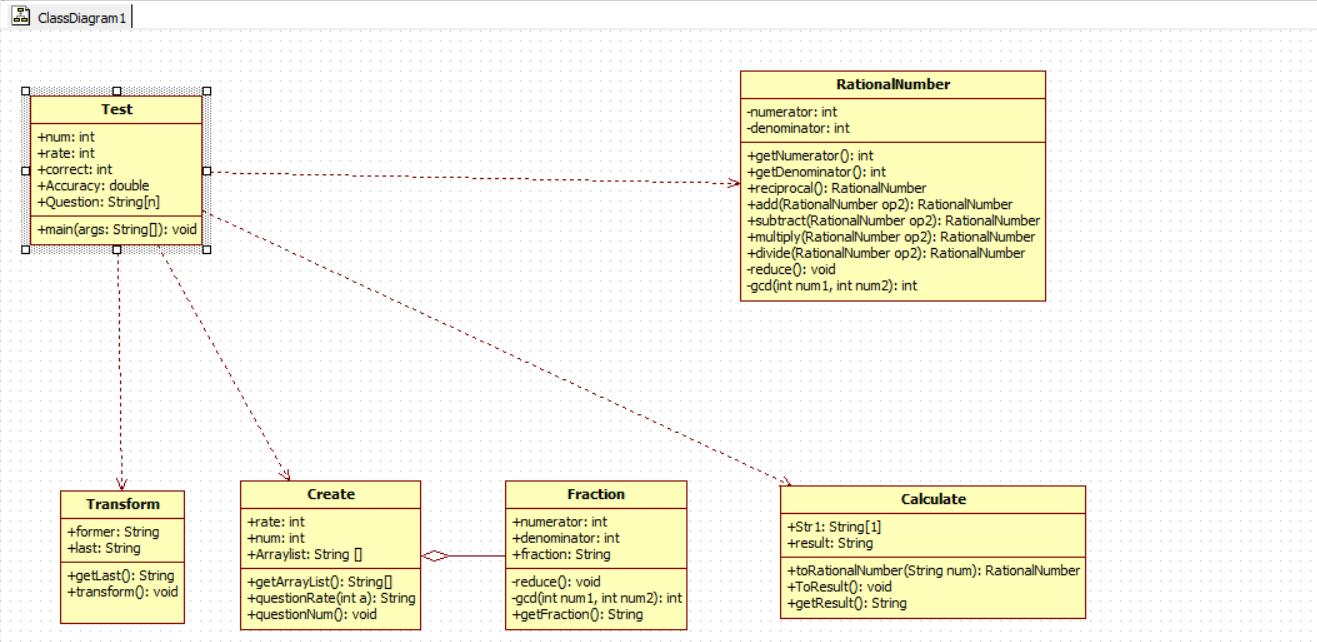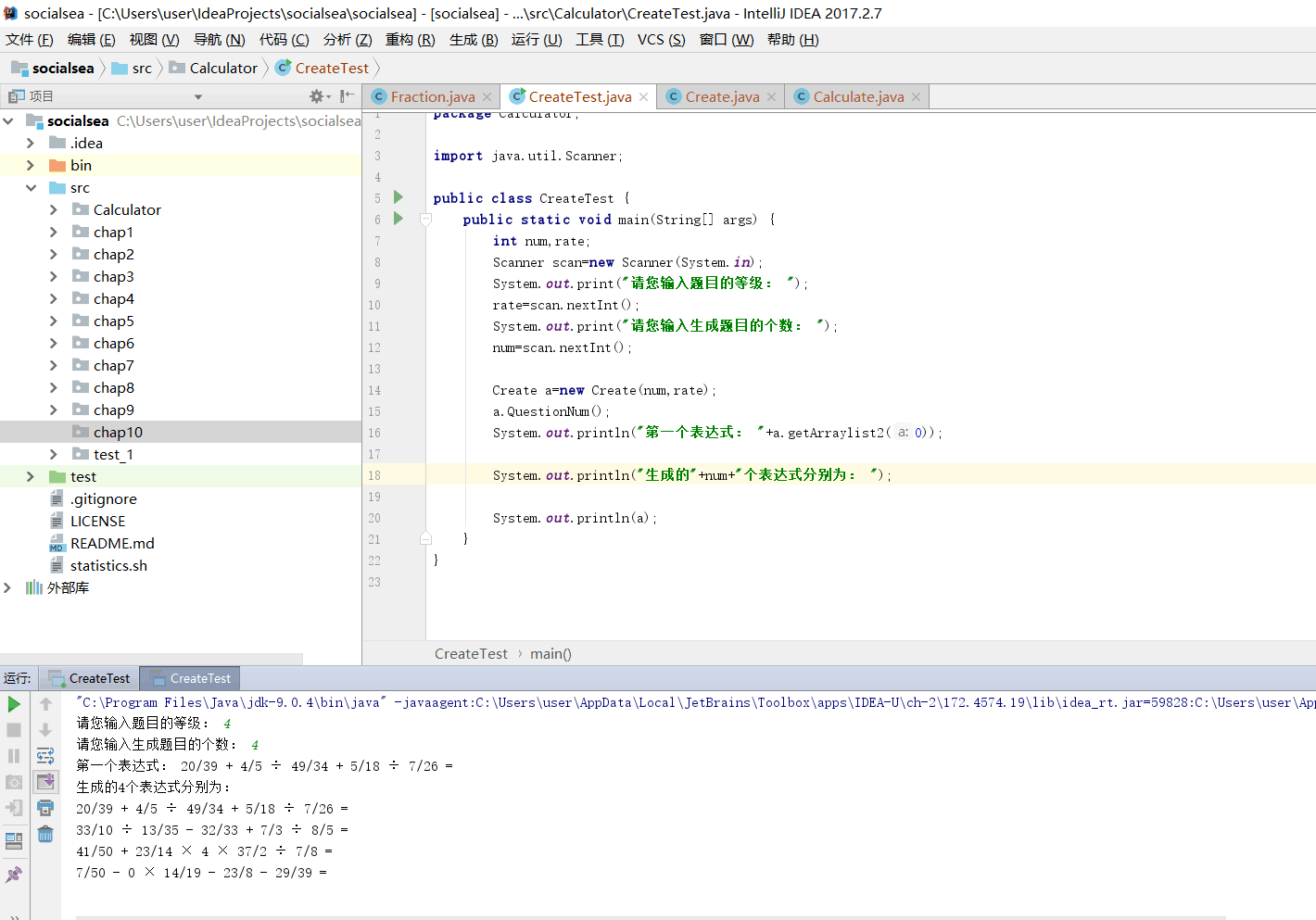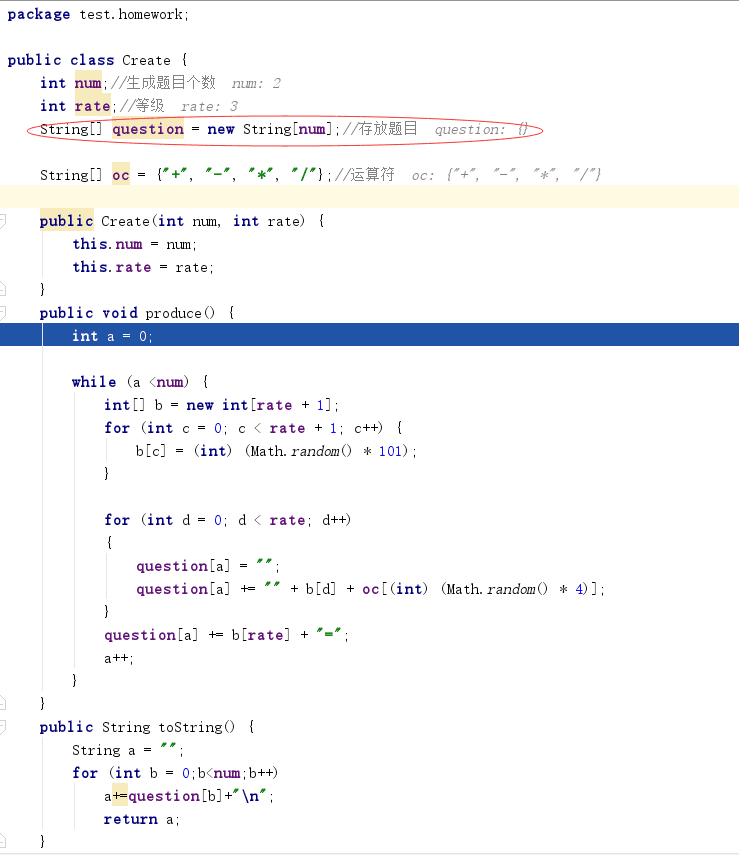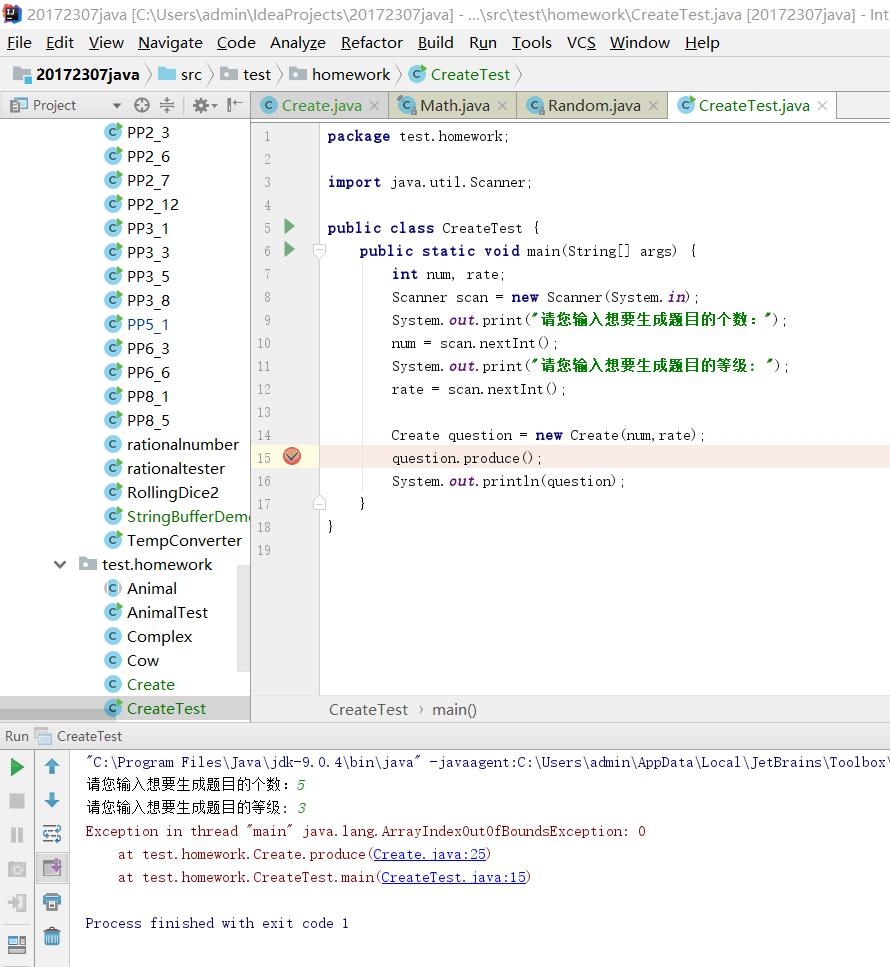# 20172311『Java程序设计』课程 结对编程练习_四则运算第一周阶段总结

• 学号 ：20172307

• 姓名 ：黄宇瑭

## 需求分析

#### <一>功能要求

1.自动生成题目

• 可独立使用（能实现自己编写测试类单独生成题目的功能）

• 可生成不同等级题目，类似于：

1级题目：2 + 5 =；
10 - 5 =
之类的两个数，一个运算符的题目

2.题目运算（判题）

• 可独立使用

• 实现中缀表达式转为后缀表达式并计算

• 判断用户答题正误，并输出正确结果

3.支持真分数

• 可独立使用

• 实现分数算式的计算

3.题目去重（扩展需求，加分项）

• 可独立使用

• 实现对自动生成表达式的去重:如下

若生成：2 + 5 =；
5 + 2 =
为同一题目

#### <二>对需求的理解

1.要有一个生成随机题目的类，题目中的数可能分数，也可能是整数。 2.要有一个将中缀表达式转化为后缀表达式的类
3.要有一个计算后缀表达式的类
4.要有一个判断题目的类
5.将以上类进行组合写出产品代码

## 设计思路

• NML类图• 思路:
要有一个能够生成随机最简分数的类，并将它运用到生成随机题目的类中去，同时生成的题目中数字和运算符之间要有一个空格符，方便在转换为后缀表达式的时候使用栈。最后要实现交互，即让客户回答问题，然后系统判断是否正确并告诉客户。

## 相关过程截图

• 生成随机最简分数的类Fraction
public class Fraction {
int numerator, denominator;

public Fraction() {
numerator= (int) (Math.random()*51);
denominator= (int) (Math.random()*51);
if(denominator==0)
denominator=1;
reduce();
}

public void reduce() {
if (numerator != 0) {
int common = gcd(Math.abs(numerator), denominator);

numerator = numerator / common;
denominator = denominator / common;
}
}

private int gcd(int num1, int num2) {
while (num1 != num2)
if (num1 > num2)
num1 = num1 - num2;
else
num2 = num2 - num1;

return num1;
}
public String getFraction()
{
String result;
if(numerator==0)
result="0";
else
if(denominator==1)
result=numerator+"";
else
result=numerator+"/"+denominator;
return result;
}
}


• 生成相应个数表达式的类Create
public class Create {
String[]Arraylist;
int num,rate;
public Create(int num ,int rate)
{
this.num=num;
this.rate=rate;
Arraylist=new String[num];
}
public String questionRate(int a)
{
String express="";
String[]OC={"+","-","×","÷"};

for (int c=0;c<a;c++) {
Fraction b = new Fraction();
String d=b.getFraction();
String e=OC[(int) (Math.random() * 4)];
while (d=="0"&&e=="÷") {
Fraction f=new Fraction();
d = f.getFraction();

}
express +=d+" "+e+ " ";
}

Fraction c=new Fraction();
String e=c.getFraction();
while (express.charAt(4*rate-1)=='÷'&&e=="0")
{
Fraction d=new Fraction();
e=d.getFraction();
}

express+=e+" "+"=";
return express;
}
public  void QuestionNum(){
Create F=new Create(num,rate);
for(int a=0;a<num;a++)
Arraylist[a]=F.questionRate(rate);
}

public String[] getArraylist() {
return Arraylist;
}
public String getArraylist2(int a)
{
String b;
b=Arraylist[a];
return b;
}

public String toString() {
String a="";
for (int b=0;b<num;b++)
a+=Arraylist[b]+"\n";
return a;
}
}


• 测试类CreateTest运行截图## 遇到的困难及解决方法

• 刚开始编写用于生成整数类型的题目的类时出现逻辑错误，导致运行测试类时出现越界。

• 错误代码截图如下：• 测试类运行结果如下：• 改过后的代码截图如下：• 错因分析：错误代码中红圈标记的区域便是错误所在，该语句创建了一个空数组，与本意创建一个存放num个String型表达式的数组相违背。从而出现越界的现象。而改过之后的代码中是在构造函数中对数组进行了实例化，也就达到目的了。

## PSP时间统计

PSP2.1 Personal Software Process Stages 预估耗时（分钟） 实际耗时（分钟）
Planning 计划 60 70
Estimate 估计这个任务需要多少时间 30 30
Development 开发 600 700
Analysis 需求分析 (包括学习新技术) 120 180
Coding Standard 代码规范 (为目前的开发制定合适的规范) 30 20
Design UML 设计项目UML类图 60 90
Coding 具体编码 180 210
Code Review 代码复审 180 120
Test 测试（自我测试，修改代码，提交修改） 120 120
Size Measurement 计算工作量（实际时间 30 20
Postmortem & Process Improvement Plan 事后总结, 并提出过程改进计划 30 60

posted on 2018-05-01 15:31  socialsea  阅读(303)  评论(2编辑  收藏  举报